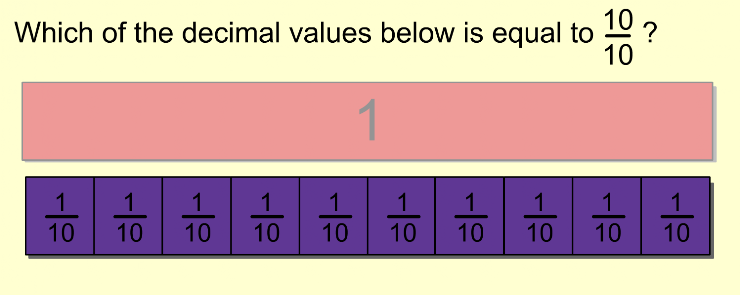Fractions and Decimals 1
Set 1 (10 Questions)
Students know and understand that fractions and decimals are two different representations of the same concept to show equivalency, and to refer to parts of a set and parts of a whole. Students can provide examples such as "50 cents is 1/2 dollar, 75 cents is 3/4 of a dollar", etc...
From Mr. Anker Tests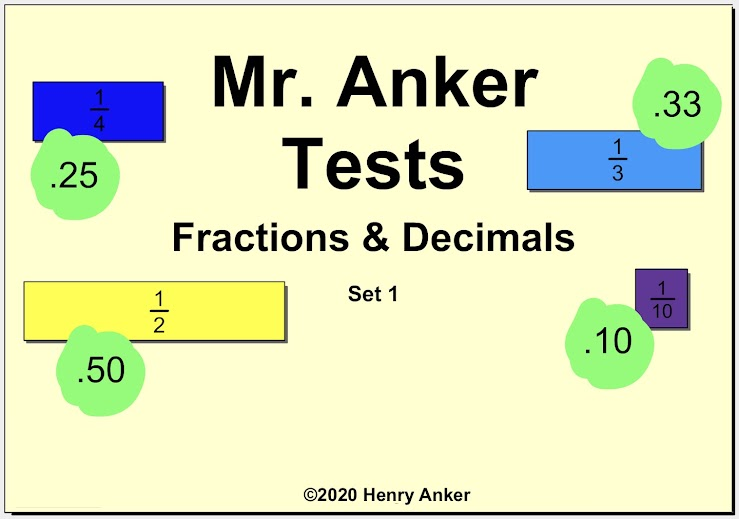Fractions & Decimals Help Slide 1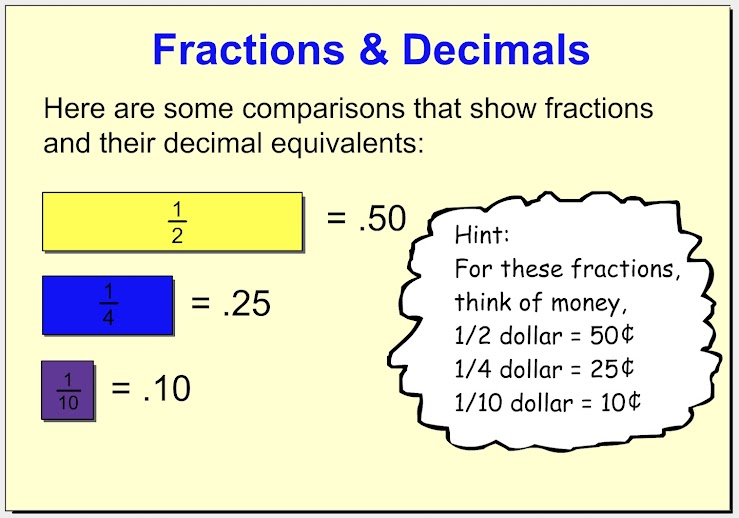Fractions & Decimals Help Slide 2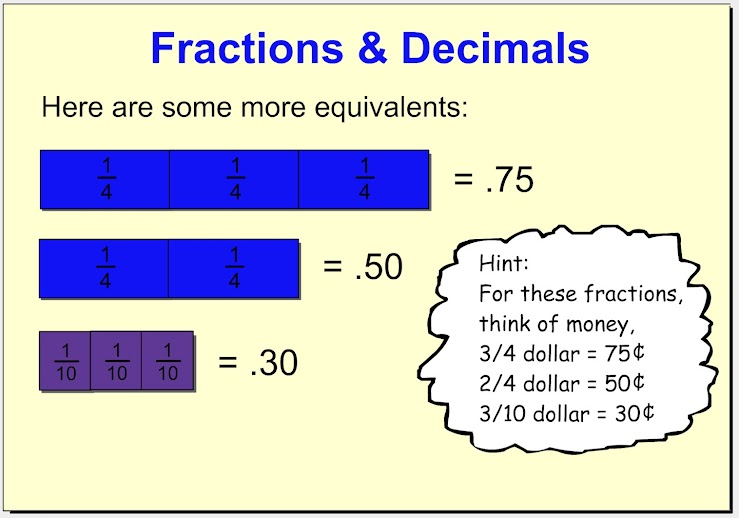1. Which of decimal values below is equal to 1/2? *
1 point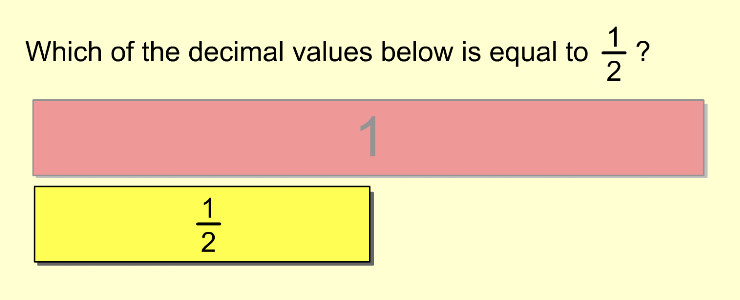2. Which of decimal values below is equal to 1/4? *
1 point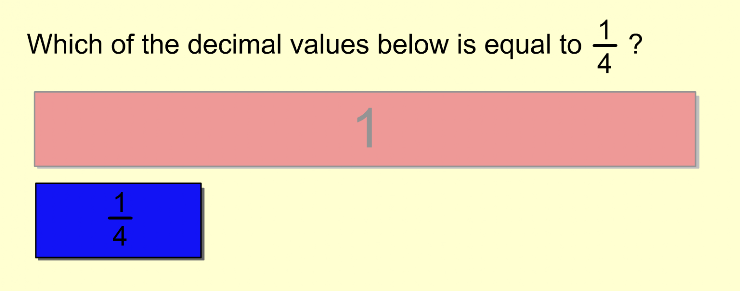3. Which of decimal values below is equal to 1/10? *
1 point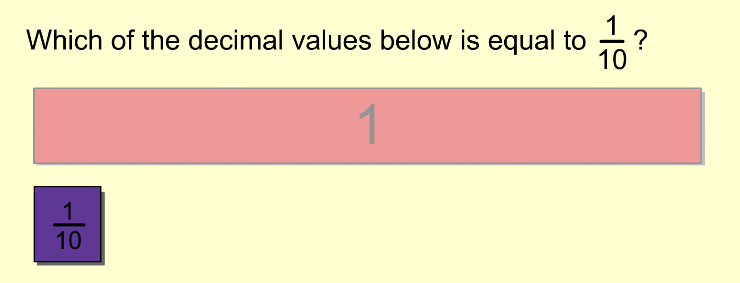4. Which of decimal values below is equal to 3/4? *
1 point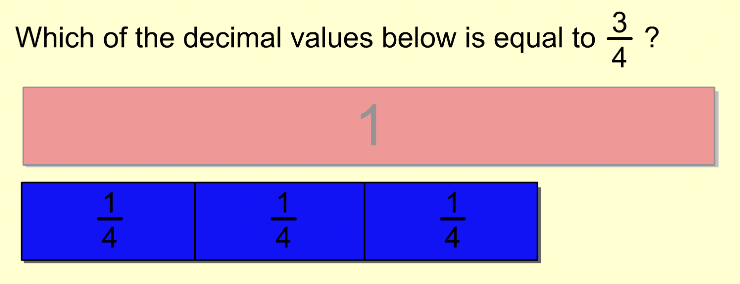5. Which of decimal values below is equal to 3/10? *
1 point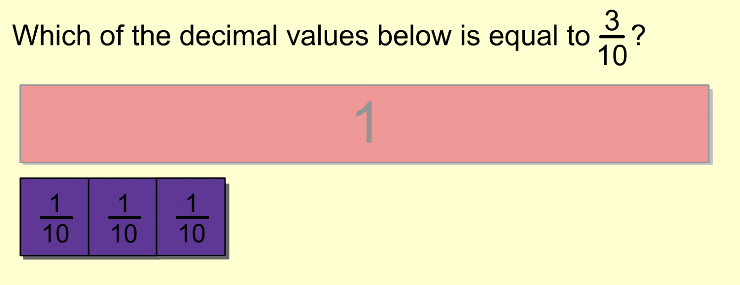6. Which of the decimal values below is equal to 3/4? *
1 point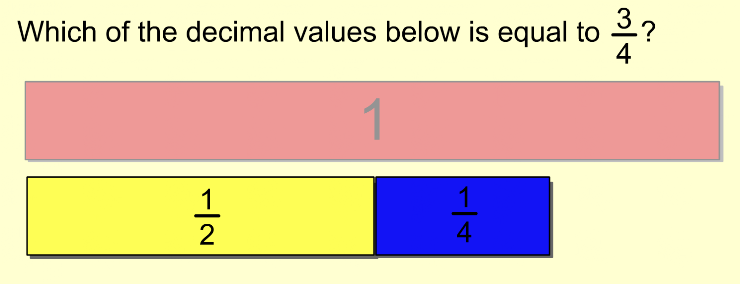7. Which of the decimal values below is equal to 6/10? *
1 point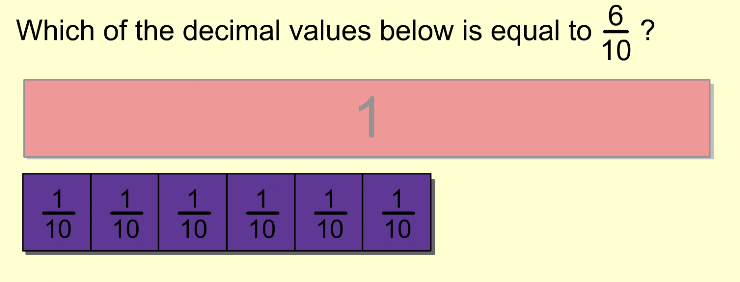8. Which of decimal values below is equal to 8/10? *
1 point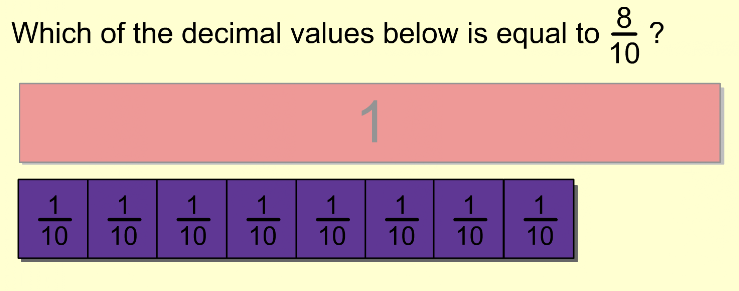9. Which of decimal values below is equal to 1/3? *
1 point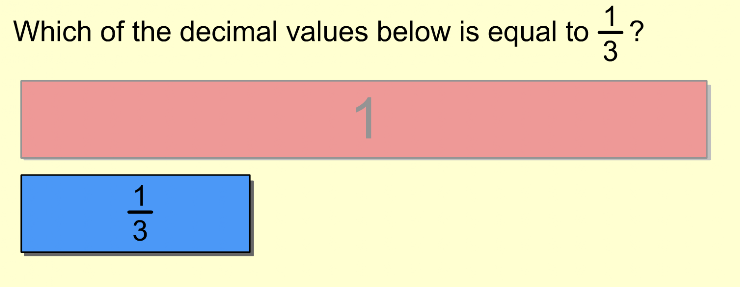10. Which set of decimal values below is equal to 1/10? *
1 point